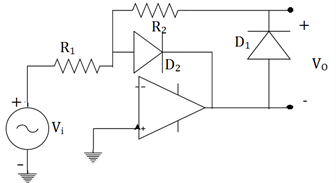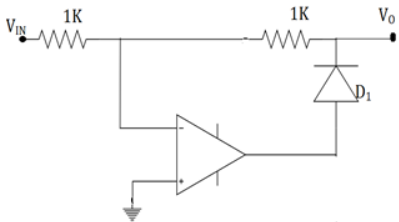Courses

# Test: Half-Wave & Full-wave Rectifier

## 20 Questions MCQ Test Electronic Devices | Test: Half-Wave & Full-wave Rectifier

Description
This mock test of Test: Half-Wave & Full-wave Rectifier for Electrical Engineering (EE) helps you for every Electrical Engineering (EE) entrance exam. This contains 20 Multiple Choice Questions for Electrical Engineering (EE) Test: Half-Wave & Full-wave Rectifier (mcq) to study with solutions a complete question bank. The solved questions answers in this Test: Half-Wave & Full-wave Rectifier quiz give you a good mix of easy questions and tough questions. Electrical Engineering (EE) students definitely take this Test: Half-Wave & Full-wave Rectifier exercise for a better result in the exam. You can find other Test: Half-Wave & Full-wave Rectifier extra questions, long questions & short questions for Electrical Engineering (EE) on EduRev as well by searching above.
QUESTION: 1

### The diode in a half wave rectifier has a forward resistance RF. The voltage is Vmsinωt and the load resistance is RL. The DC current is given by _________

Solution:

For a half wave rectifier, the IDC=IAVG=Im
I= Vmsinωt/(RF+RL)=Imsinωt
Im =Vm/ RF+RL So, IDC=Im/π=Vm/(RF+RL).

QUESTION: 2

### The below figure arrives to a conclusion that _________Solution:

The given op-amp is in inverting mode and this makes the output voltage to have a phase shift of 180°. The output voltage is now negative. So, the diode 1 is reverse biased and diode 2 is forward biased. Then output is clearly zero.

QUESTION: 3

### What is the output as a function of the input voltage (for positive values) for the given figure. Assume it’s an ideal op-amp with zero forward drop (Di=0)Solution:

When the input of the inverted mode op-amp is positive, the output is negative.
The diode is reverse biased. The input appears at the output.

QUESTION: 4

In a half wave rectifier, the sine wave input is 50sin50t. If the load resistance is of 1K, then average DC power output will be?

Solution:

The standard form of a sine wave is Vmsinωt. BY comparing the given information with this equation, Vm =50.
Power=Vm2/RL=50*50/1000=2.5V.

QUESTION: 5

In a half wave rectifier, the sine wave input is 200sin300t. The average value of output voltage is?

Solution:

Comparing with the standard equation, Vm=200V.
Average value is given by, Vavg=Vm/π.
So, 200/π=63.694.

QUESTION: 6

Efficiency of a half wave rectifier is

Solution:

Efficiency of a rectifier is the effectiveness to convert AC to DC. For half wave it’s 40.6%. It’s given by, Vout/Vin*100.

QUESTION: 7

If peak voltage for a half wave rectifier circuit is 5V and diode cut in voltage is 0.7, then peak inverse voltage on diode will be?

Solution:

PIV is the maximum reverse bias voltage that can be appeared across a diode in the given circuit, If the PIV rating is less than this value of breakdown of diode will occur. For a rectifier, PIV=Vm-Vd=5-0.7=4.3V.

QUESTION: 8

Transformer utilisation factor of a half wave rectifier is _________

Solution:

Transformer utilisation factor is the ratio of AC power delivered to load to the DC power rating. This factor indicates effectiveness of transformer usage by rectifier. For a half wave rectifier, it’s low and equal to 0.287.

QUESTION: 9

If the input frequency of a half wave rectifier is 100Hz, then the ripple frequency will be_________

Solution:

The ripple frequency of the output and input is same. This is because, one half cycle of input is passed and other half cycle is seized. So, effectively the frequency is the same.

QUESTION: 10

Ripple factor of a half wave rectifier is_________(Im is the peak current and RL is load resistance)

Solution:

The ripple factor of a rectifier is the measure of disturbances produced in the output. It’s the effectiveness of a power supply filter to reduce the ripple voltage. The ratio of ripple voltage to DC output voltage is ripple factor which is 1.21.

QUESTION: 11

Efficiency of a centre tapped full wave rectifier is _________

Solution:

Efficiency of a rectifier is the effectiveness to convert AC to DC. It’s obtained by taking ratio of DC power output to maximum AC power delivered to load. It’s usually expressed in percentage. For centre tapped full wave rectifier, it’s 81.2%.

QUESTION: 12

A full wave rectifier supplies a load of 1KΩ. The AC voltage applied to diodes is 220V (rms). If diode resistance is neglected, what is the ripple voltage?

Solution:

The ripple voltage is (Vϒ)RMS=ϒVDC /100.
VDC=0.636*VRMS* √2=0.636*220* √2=198V and ripple factor ϒ for full wave rectifier is 0.482.
Hence, (Vϒ)RMS=0.482*198 /100=0.954V.

QUESTION: 13

A full wave rectifier delivers 50W to a load of 200Ω. If the ripple factor is 2%, calculate the AC ripple across the load.

Solution:

We know that, PDC=VDC2/RL. So, VDC=(PDC*RL)1/2=100001/2=100V.
Here, ϒ=0.02
ϒ=VAC/VDC=VAC/100.So, VAC=0.02*100=2V.

QUESTION: 14

A full wave rectifier uses load resistor of 1500Ω. Assume the diodes have Rf=10Ω, Rr=∞. The voltage applied to diode is 30V with a frequency of 50Hz. Calculate the AC power input.

Solution:

The AC power input PIN=IRMS2(RF+Rr).
IRMS=Im/√2=Vm/(Rf+RL)√2=30/(1500+10)*1.414=13.5mA
So, PIN=(13.5*10-3)2*(1500+10)=275.2mW.

QUESTION: 15

In a centre tapped full wave rectifier, RL=1KΩ and for diode Rf=10Ω. The primary voltage is 800sinωt with transformer turns ratio=2. The ripple factor will be _________​Solution:

The ripple factor ϒ= [(IRMS/IAVG)2 – 1]1/2. IRMS =Im /√2=Vm/(Rf+RL)√2=200/1.01=198.
(Secondary line to line voltage is 800/2=400. Due to centre tap Vm=400/2=200)
IRMS=198/√2=140mA, IAVG=2*198/π=126mA. ϒ=[(140/126)2-1]1/2=0.48. So, ϒ=48%.

QUESTION: 16

If input frequency is 50Hz for a full wave rectifier, the ripple frequency of it would be _________

Solution:

In the output of the centre tapped rectifier, one of the half cycle is repeated. The frequency will be twice as that of input frequency. So, it’s 100Hz.

QUESTION: 17

Transformer utilization factor of a centre tapped full wave rectifier is_________

Solution:

Transformer utilisation factor is the ratio of AC power delivered to load to the DC power rating. This factor indicates effectiveness of transformer usage by rectifier. For a half wave rectifier, it’s low and equal to 0.693.

QUESTION: 18

In the circuits given below, the correct full wave rectifier is _________

Solution:

When the input is applied, a full wave rectifier should have a current flow. The flow should be in the same direction for both positive and negative half cycles. Only the third circuit satisfies the above condition.

QUESTION: 19

If the peak voltage on a centre tapped full wave rectifier circuit is 5V and diode cut in voltage is 0.7. The peak inverse voltage on diode is_________

Solution:

PIV is the maximum reverse bias voltage that can be appeared across a diode in the given circuit, if PIV rating is less than this value of breakdown of diode will occur. For a rectifier, PIV=2Vm-Vd = 10-0.7 = 9.3V.

QUESTION: 20

In a centre tapped full wave rectifier, the input sine wave is 250sin100t. The output ripple frequency will be _________

Solution:

The equation of sine wave is in the form Vmsinωt. So, by comparing we get ω=100. Frequency, f =ω/2=50Hz. The output of centre tapped full wave rectifier has double the frequency of inpu. Hence, fout = 100Hz.